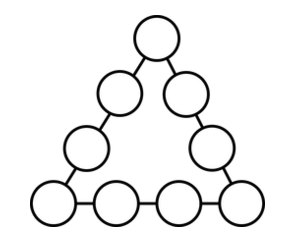Home > Grade 7 > Geometry > Equilateral Triangle

# Equilateral Triangle

Directions: Using the whole numbers 1 through 9, no more than one time each, fill in the circles of the triangle. The sum of the numbers on each side of the triangle is equal to the length of that side. Arrange the numbers so that the triangle is an equilateral triangle.### Hint

What are the criteria for an equilateral triangle?